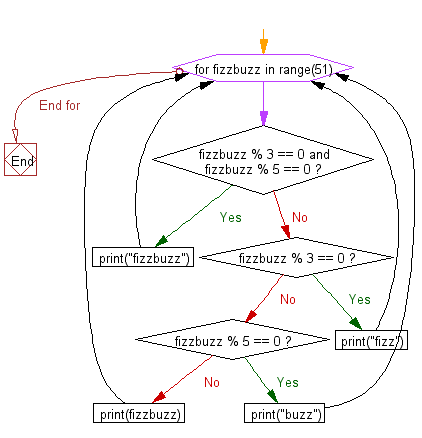﻿ Python Exercise: Get Fizz, Buzz and FizzBuzz - w3resource# Python Exercise: Get Fizz, Buzz and FizzBuzz

## Python Conditional: Exercise-10 with Solution

Write a Python program which iterates the integers from 1 to 50. For multiples of three print "Fizz" instead of the number and for the multiples of five print "Buzz". For numbers which are multiples of both three and five print "FizzBuzz".

Sample Solution:

Python Code :

``````for fizzbuzz in range(51):
if fizzbuzz % 3 == 0 and fizzbuzz % 5 == 0:
print("fizzbuzz")
continue
elif fizzbuzz % 3 == 0:
print("fizz")
continue
elif fizzbuzz % 5 == 0:
print("buzz")
continue
print(fizzbuzz)
```
```

Sample Output:

```fizzbuzz
1
2
fizz
4
buzz
fizz
7
8
fizz
buzz
11
fizz
13
14
fizzbuzz
.....

41
fizz
43
44
fizzbuzz
46
47
fizz
49
buzz
```

Flowchart:## Visualize Python code execution:

The following tool visualize what the computer is doing step-by-step as it executes the said program:

Python Code Editor :

Have another way to solve this solution? Contribute your code (and comments) through Disqus.

What is the difficulty level of this exercise?

Test your Python skills with w3resource's quiz

﻿

## Python: Tips of the Day

Returns a list with n elements removed from the beginning

Example:

```def tips_take(itr, n = 1):
return itr[:n]
print(tips_take([1, 2, 3], 5))
print(tips_take([1, 2, 3], 0))
```

Output:

```[1, 2, 3]
[]
```